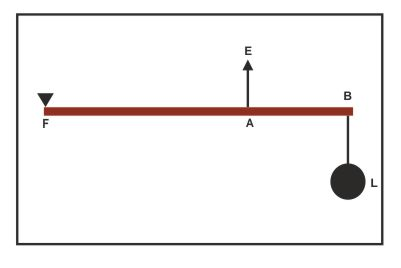Class III Levers - Physics
23-11-2018    188 timesIn these types of levers, the fulcrum F and the Load (L) are at the two ends of levers and the effort (E) is somewhere in between the fulcrum F and the load(L) as shown in figure. The effort and load are work in the opposite directions and the effort arm is always smaller than the load arm. Therefore form the equation M.A < 1 and since for an ideal lever M.A. is equal to V.R

In fig, BF is a class III lever in this lever F is the fulcrum and L is the load at the point B. the effort E act on the point A. here BF is the load arm and AF is the effort arm. Here, BF>AF.

E × AF =  L × BF

Mechanical advantage = (L/E)=(AF/BF)

The mechanical advantage and velocity ratio of class II levers are always less than 1.

### Example Of Class III leverQuery About the post